# Build Junction Tree for BN.docx

The note is to generate the Junction Tree step by step, with two examples, one is simple BN, another is complex BN.

1. Reference:
1. PGM_CS 731 Advanced methods in artificial intelligence, with biomedical applications (Fall 2009): http://pages.cs.wisc.edu/~dpage/cs731/lecture5.ppt
2. Text: Bayesian Reasoning and Machine Learning
3. https://en.wikipedia.org/wiki/Kruskal%27s_algorithm

1. Sample 1:

Below is a full procedure on how to generate a Junction tree with complete potential and separators from simple Bayes model

1. Problem: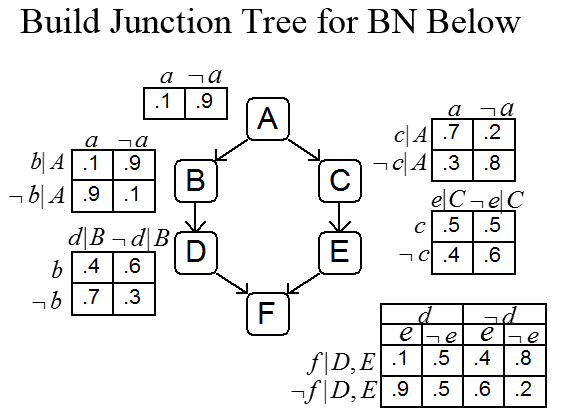1. Turn it into Cliques graph: moralized, and triangulated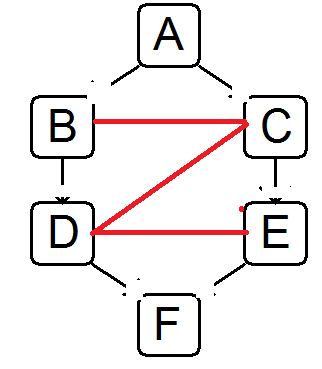Here is the cliques set:   {ABC}   {BCD}  {CDE}  {DEF}

1.  Turn it into a Junction Tree:

Make sure it complies with JTP, Junction tree property: For each pair U, V of cliques with intersection S, all cliques on the path between U and V contain S.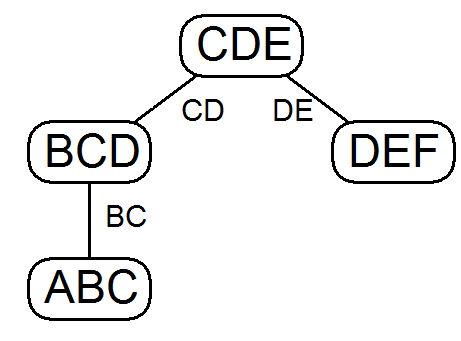1. BN formula

From original BN,It can rewrite as local cliques marginal format:(0)

1. Populate Clique Nodes:  Pick CDE as root
• For each distribution (CPT) in the original Bayes Net, put this distribution into one of the clique nodes that contains all the variables referenced by the CPT.  (At least one such node must exist because of the moralization step).
• For each clique node, take the product of the distributions (as in variable elimination).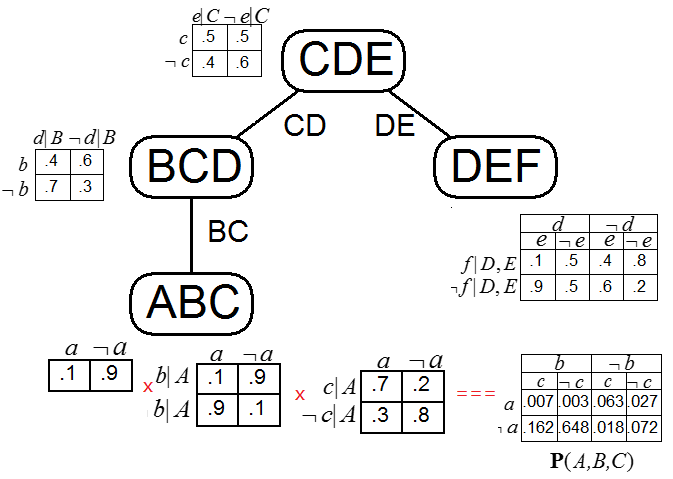(1)

1.  Let’s start to compute the Separator and Cliques for the upward procedure, start from ABC cliques.

1.=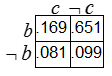(sum two rows of a and ~a in P(A,B,C) in above diagram (1))
2.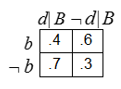X=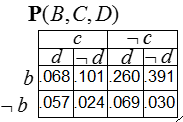(2)

1.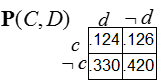(that is sum two rows of b and ~b in P(B,C,D) in above formula (2))
2. [Image_Link]https://lh5.googleusercontent.com/R0QWQ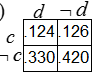x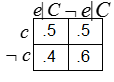=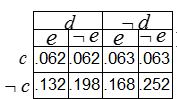1. After the Upward step, the full diagram with updated distribution as below: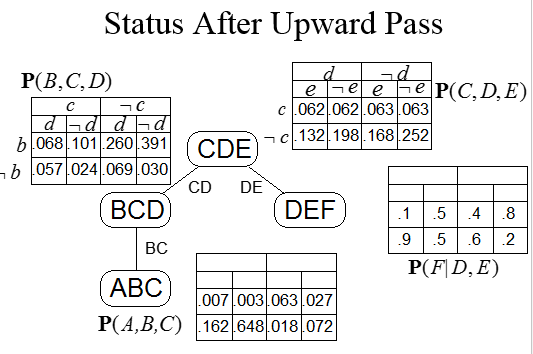1. Let’s do the downward step:
1.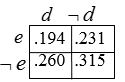2.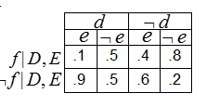X=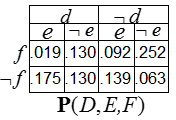1. After that, we finish all Cliques and Separator distributions update:

From the below diagram, you can see the graph is represent the joint distribution formula (0).  It contains the result of subset of marginal, P(BC), P(ABC), etc.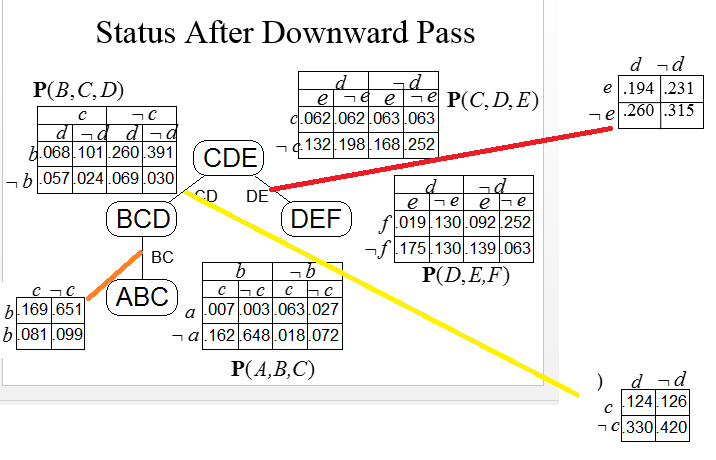1. Further for evidence:

If you observe evidence, you need to Incorporate Evidence before do the above upward and down steps to generate clique’s distribution.

• Incorporate Evidence: For each evidence variable, go to one table that includes that variable.  Set to 0 all entries in that table that disagree with the evidence.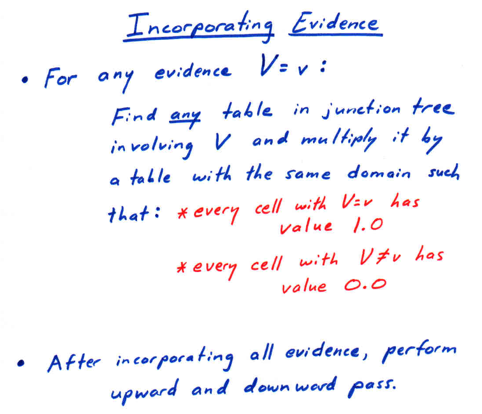For example, in the first step to populate the distribution, if we know e=1, we need to update to update the original distribution, for example the CDE cliques in the diagram (1).

P(CDE)=x=Here is the summary for evidence.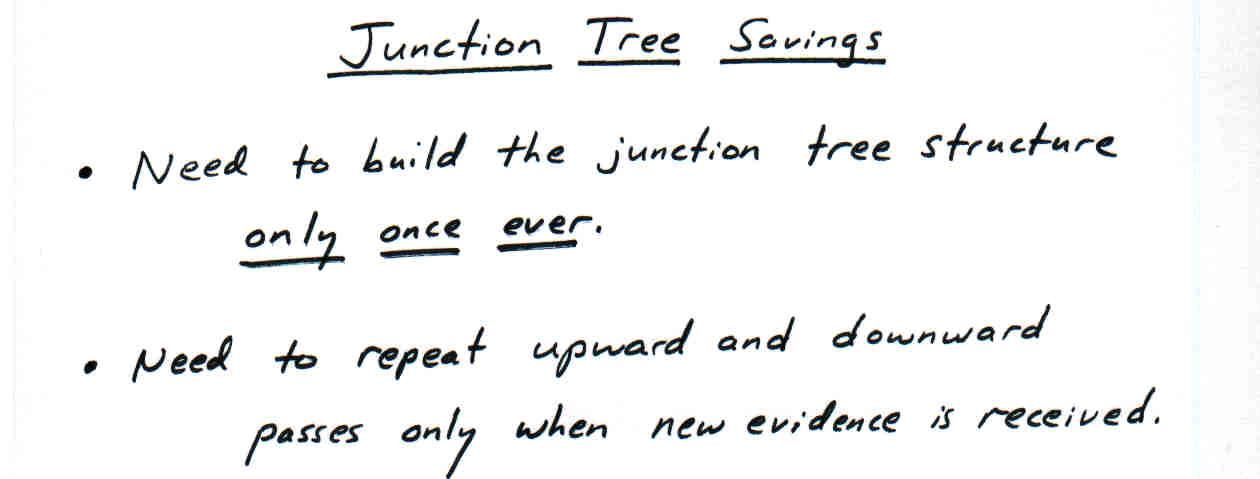1.  Sample 2.

Below is a full procedure on how to generate a Junction tree from complex Bayes model

1. original Bayes model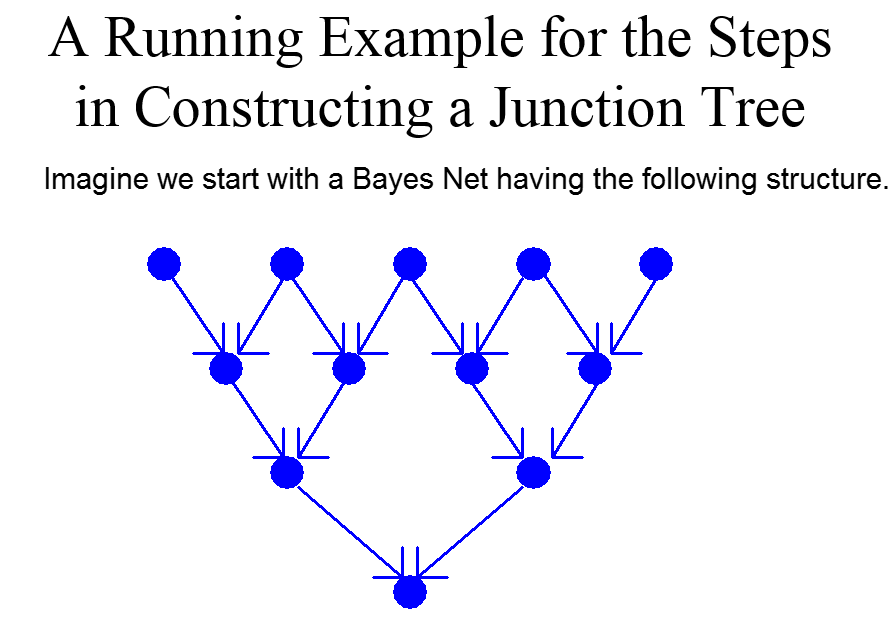1.  Moralize directed graph: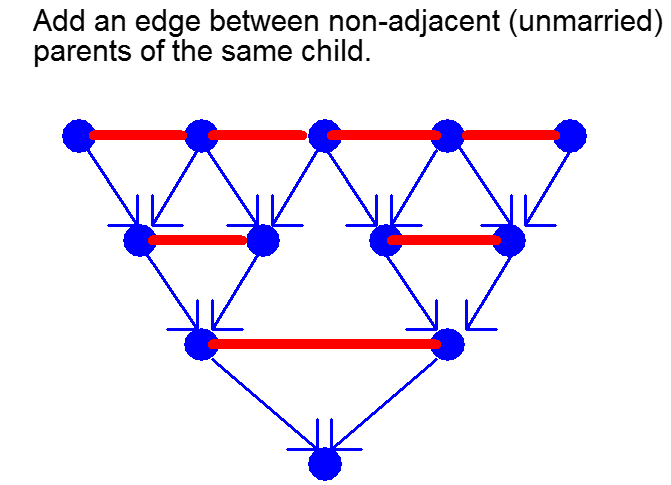1. Triangulate an undirected graph:

Textbook “Bayesian Reasoning and Machine Learning_2017Version” provides the detail example too on how to form a triangulated clique graph:

P114: Figure 6.8:

1. First drop the simplicial node is one whose neighbors form a clique: every two neighbors are adjacent.
2. Drop the edge also when drop a node.
3. Drop any other node, if its two adjacent nodes cannot connected, add an edge between the two nodes.
4. Repeat 1 and 2, until no more node delete.
5. Below is example, from left to right, top to bottom on drop a node.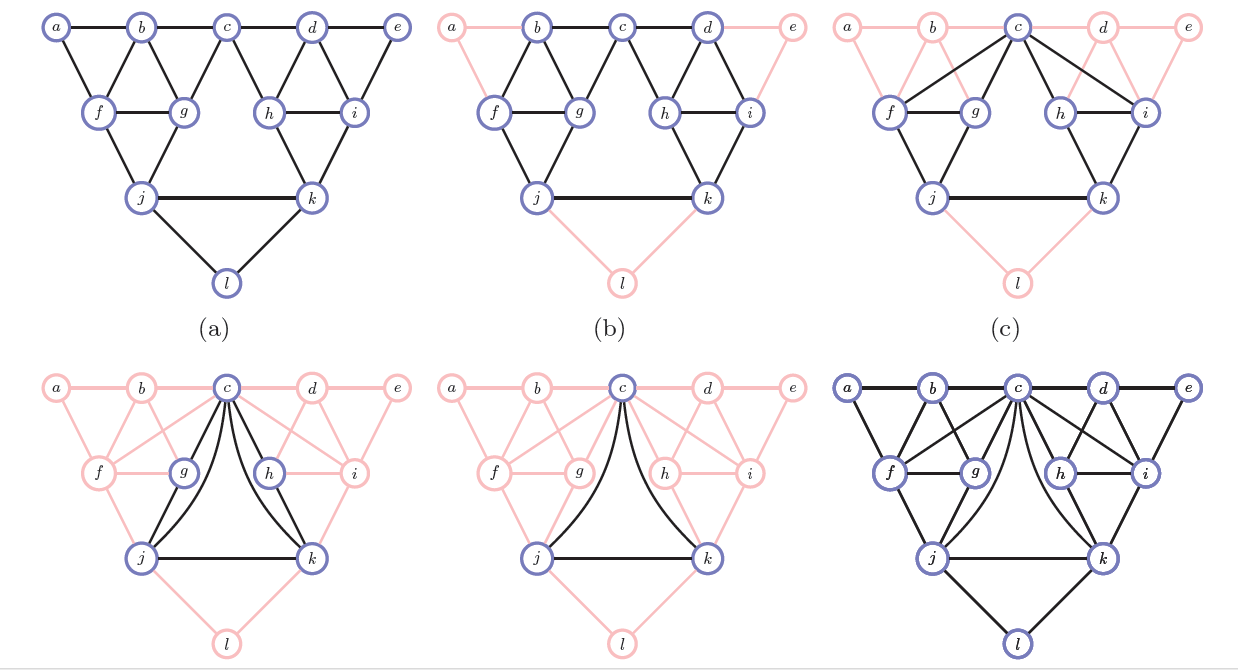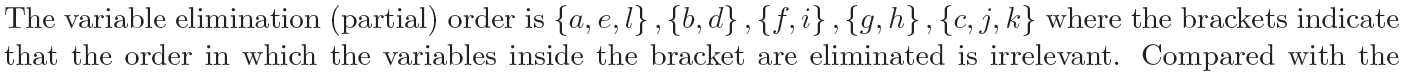You can ref to textbook P115, Algorithm 6.1 to check whether to the above last triangulated diagram (bottom right) is already triangulated.

Algorithm 6.1: Check if a graph is decomposable (triangulated).

The graph is triangulated if, after cycling through all the n nodes in the graph, the FAIL criterion is not encountered.

1.  Choose any node in the graph and label it 1.
2.  for i = 2 to n do
3.  Choose the node with the most labeled neighbors and label it i.
4.  If any two labeled neighbors of i are not adjacent to each other, FAIL.
5.  end for

Where there is more than one node with the most labeled neighbors, the tie may be broken arbitrarily.

1. Continue to build a Junction Tree according to the above last triangulated diagram (bottom right).

We are using the Kruskal’s minimum spanning tree algorithm, by multiply edge weight with -1.   The edge weight is the number of variables in the separator between cliques i and j.

You can refer the animation here: https://en.wikipedia.org/wiki/Kruskal%27s_algorithm

Below diagram is from “PGM_CS 731 Advanced methods in artificial intelligence, with biomedical applications (Fall 2009)) lecture5.ppt” http://pages.cs.wisc.edu/~dpage/cs731/lecture5.ppt

*a) relabel the above last diagram as: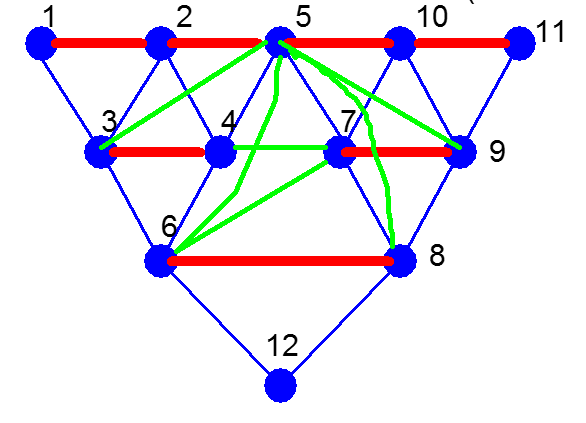*b) generate the clique graph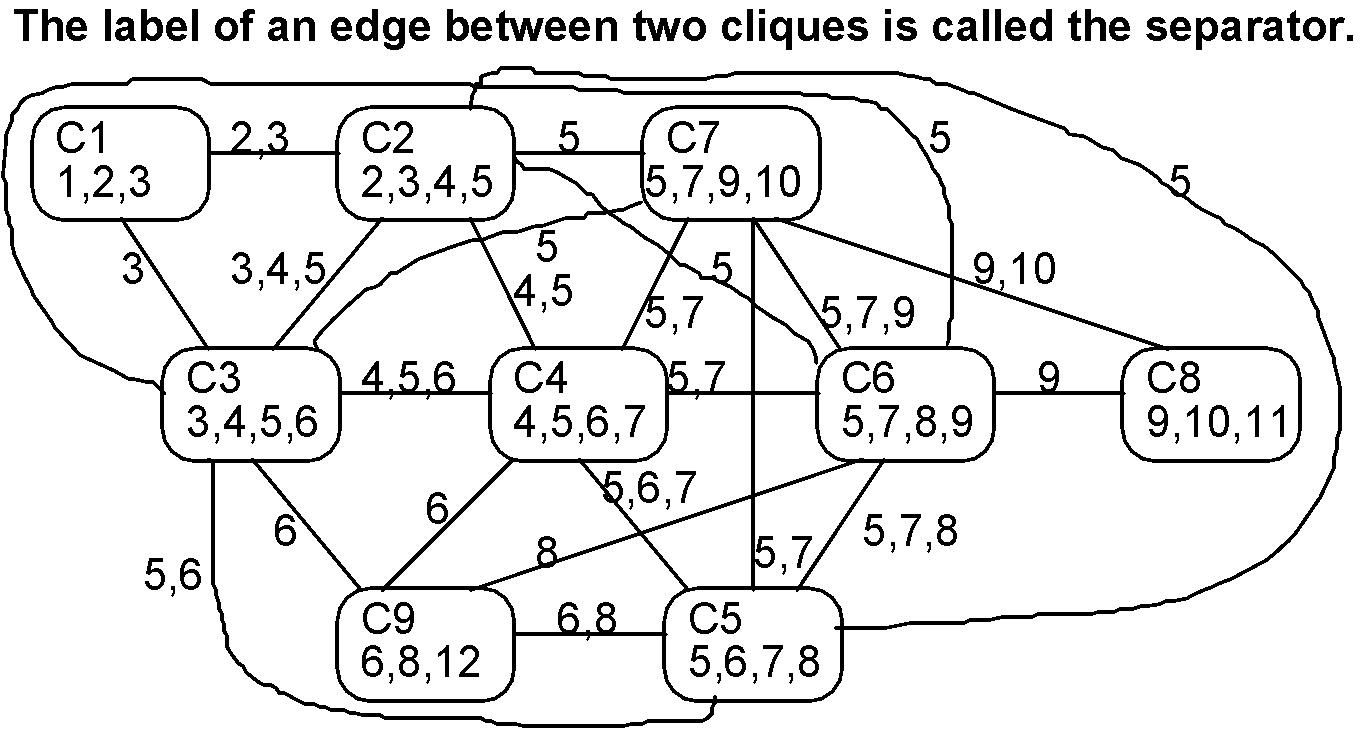*c) using Kruskal’s minimum spanning tree get the get span tree

Assign weight as number of separators multiply -1: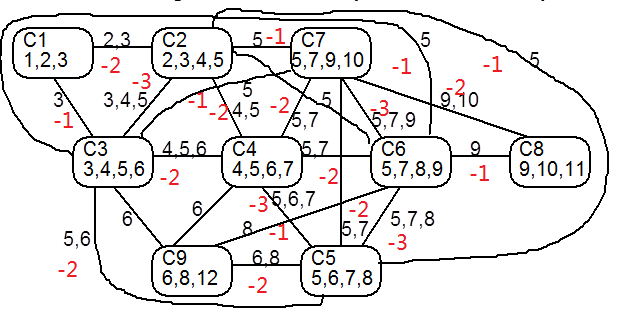After that, get the minimum edge first, then continue, if next minimum edge form a cycle, then do not select the minimum edge, repeated until all cliques are included. So here is the sequence:

Weight=-3:

C7 N C6; C6 N C5; C5 N C4; C4 N C3; C3 N C2;

Weight=-2:

C7 N C8; C2 N C1; C5 N C9

Here is the final Junction Tree result: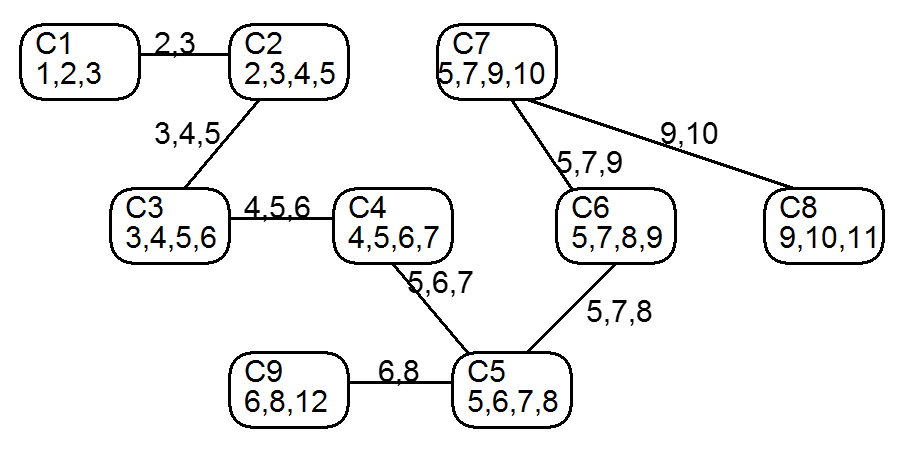Make it beautify and balance, you got the final version of Junction tree.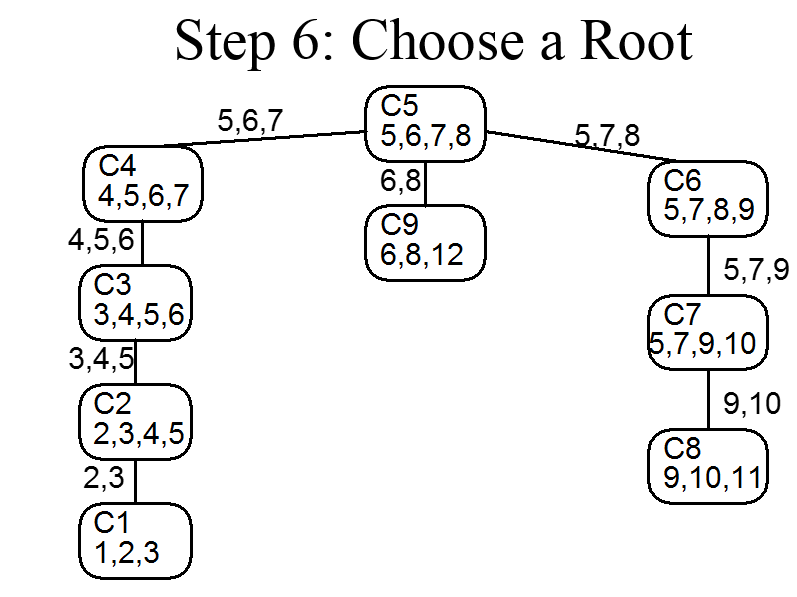After that, you can use 1. Sample 1 on how to specify the complete potential and separators.